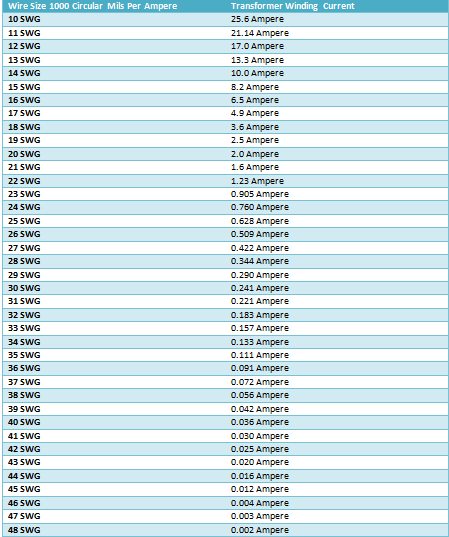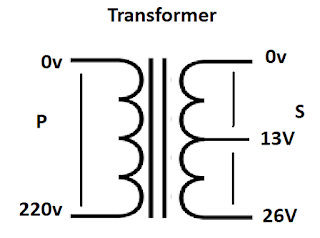## Arduino Relay Control with 433 MHz RF Remote and Receiver Connection and Coding

This is post is about the arduino 4 relay control module which control using the 433 MHz remote control module and receiver. Or how to connect the RF 433mHZ with arduino for remote controlling or debounce system. In this post I am going to share the complete sketch code is used for controlling debounce led or relay.

The below is complete sketch code for debounce. Just copy and paste. Verify the code and click on upload. You can change the setting or do changing in code according your needs.

// set pin numbers:
const int buttonPin = 2;    // the number of the pushbutton pin
const int ledPin = 12;      // the number of the LED pin
const int buttonPin2 = 3;    // the number of the pushbutton pin
const int ledPin2 = 11;      // the number of the LED pin
const int buttonPin3 = 4;
const int ledPin3 = 10;
const int buttonPin4 = 5;
const int ledPin4 = 9;
// Variables will change:
int ledState = HIGH;         // the current state of the output pin
int buttonState;             // the current reading from the input pin
int lastButtonState = LOW;   // the previous reading from the input pin
int ledState2 = HIGH;         // the current state of the output pin
int buttonState2;             // the current reading from the input pin
int lastButtonState2 = LOW;   // the previous reading from the input pin
int ledState3 = HIGH;
int buttonState3;
int lastButtonState3 = LOW;
int ledState4 = HIGH;
int buttonState4;
int lastButtonState4 = LOW;

// the following variables are long's because the time, measured in miliseconds,
// will quickly become a bigger number than can be stored in an int.
long lastDebounceTime = 0;  // the last time the output pin was toggled
long debounceDelay = 50;    // the debounce time; increase if the output flickers
long lastDebounceTime2 = 0;  // the last time the output pin was toggled
long debounceDelay2 = 50; // the debounce time; increase if the output flickers
long lastDebounceTime3 = 0;
long debounceDelay3 = 50;
long lastDebounceTime4 = 0;
long debounceDelay4 = 50;

void setup() {
pinMode(buttonPin, INPUT);
pinMode(ledPin, OUTPUT);
pinMode(buttonPin2, INPUT);
pinMode(ledPin2, OUTPUT);
pinMode(buttonPin3, INPUT);
pinMode(ledPin3, OUTPUT);
pinMode(buttonPin4, INPUT);
pinMode(ledPin4, OUTPUT);

// set initial LED state
digitalWrite(ledPin, ledState);
digitalWrite(ledPin2, ledState2);
digitalWrite(ledPin3, ledState3);
digitalWrite(ledPin4, ledState4);

}

void loop() {
// read the state of the switch into a local variable:

// check to see if you just pressed the button
// (i.e. the input went from LOW to HIGH),  and you've waited
// long enough since the last press to ignore any noise:

// If the switch changed, due to noise or pressing:
// reset the debouncing timer
lastDebounceTime = millis();
}

// reset the debouncing timer
lastDebounceTime2 = millis();
}
// reset the debouncing timer
lastDebounceTime3 = millis();
}
// reset the debouncing timer
lastDebounceTime4 = millis();
}

if ((millis() - lastDebounceTime) > debounceDelay) {
if ((millis() - lastDebounceTime2) > debounceDelay2) {
if ((millis() - lastDebounceTime3) > debounceDelay3) {
if ((millis() - lastDebounceTime4) > debounceDelay4) {

// whatever the reading is at, it's been there for longer
// than the debounce delay, so take it as the actual current state:

// if the button state has changed:

// only toggle the LED if the new button state is HIGH
if (buttonState == HIGH) {
ledState = !ledState;
}
}
}

// only toggle the LED if the new button state is HIGH
if (buttonState2 == HIGH) {
ledState2 = !ledState2;
}
}
}

// only toggle the LED if the new button state is HIGH
if (buttonState3 == HIGH) {
ledState3 = !ledState3;
}
}
}

// only toggle the LED if the new button state is HIGH
if (buttonState4 == HIGH) {
ledState4 = !ledState4;
}
}
}

// set the LED:
digitalWrite(ledPin, ledState);
digitalWrite(ledPin2, ledState2);
digitalWrite(ledPin3, ledState3);
digitalWrite(ledPin4, ledState4);

// save the reading.  Next time through the loop,
// it'll be the lastButtonState:

}
The above arduino code is for switch on off the leds using button. However we use the 433MHz Wireless Remote Control Switch Module Transceiver Receiver Transmitter DC 2.5V-5.0V with arduino to switch on/off the relay and relay control the light bulb, ceiling fan, socket etc in room wiring.

Watch the video for complete understanding.

I hope after watch the video, now you able to connect the wireless remote control (433MHZ) to arduino. Now if you have any question then use the below comment section.

## Arduino Led Sequential Control Complete Guide

This post is about the Arduino led sequential control. This video is about the multiple leds blinking project with coding. All the coding and connection method is complete cover in this post with video tutorial.

## Arduino Multiple Led Sequential control, Blink led one by one

The project code for blinking led one by one using arduino. note that for output and blinking led. The arduino digital pins are used. In the below coding the digital pins 8, 9, 10, 11 are used for out put or to blink led. The led will one for 1 second and all led will off for half- second. You can change the delay time according your need.

void setup() {
// put your setup code here, to run once:
pinMode(8, OUTPUT);
pinMode (9, OUTPUT);
pinMode (10, OUTPUT);
pinMode (11, OUTPUT);
}

void loop() {
// put your main code here, to run repeatedly:
digitalWrite (8, HIGH);
delay (1000);
digitalWrite (8, LOW);
delay (500);
digitalWrite (9, HIGH);
delay (1000);
digitalWrite (9, LOW);
delay (500);
digitalWrite (10, HIGH);
delay (1000);
digitalWrite (10, LOW);
delay (500);
digitalWrite (11, HIGH);
delay (1000);
digitalWrite (11, LOW);
delay (500);
}
Just copy and past. And upload into your arduino. Watch the below video tutorial for complete understanding.

## 3 Phase Distribution Board Wiring Diagram

Hello every one... Today you will learn about the 3 phase distribution board wiring diagram. Not only a diagram but also a video tutorial in Urdu/Hindi language. So what's you need... In the today 3 phase panel board wiring diagram. You will learn about a 3 phase distribution board. In the three phase distribution board we have 6 MCCB (molded case circuit breakers). In which 600 Ampere circuit breaker as main circuit breaker. Five 100 A MCCB circuit breakers, volt meter 0 to 500 VAC, 200/5A current transformer and Ampere meter. Light indicators and earthling connection.

## 3 Phase Distribution Board Wiring Diagram

In the below Three phase panel board wiring diagram. The complete wiring shown step by step. In the diagram we use wired the MCCB circuit breakers. The all wiring done with Bus bar. In the diagram the two voltmeter wired for 3 phase voltage measuring.

(note that the Red, Yellow, Blue color is used for Line1, Line2, Line3. The black color is used for neutral and Green color is used for the earth wire.)

The current transformer is 200/5A and also same ratio of CT type ampere meter. The ampere meter class 1.5.

### Three phase Distribution board wiring Diagram explained Complete in video

For complete understanding the 3 phase panel board wiring diagram, watch the below video tutorial. In the below video tutorial I explained the complete diagram In Urdu/Hindi language.

I hope after watching the above distribution board wiring diagram video tutorial. Now you will be complete understood. However you can ask your question in  the below commenting system. Thanks for visiting and watching the video tutorial.

## How to Choose Suitable Wire Size for Transformer Winding

Today I am sharing with you the simple method of choosing the suitable wire size for transformer for winding. Or how to chose the wire size for transformer winding. To chose the suitable wire size for transformer primary winding and secondary winding is first and mine point of designing a transformer. Today I will share with you simple table which help you to select the wire size for a transformer.

As you know that we already talk about the turn per volt formula. however in this post we will also use in example to make it easy to understand. And if you would like to learn it complete then watch the below video tutorial first in Urdu/Hindi language.

## How to chose the wire size for transformer winding?

Before choosing a transformer we need to think about the some main points…
For example

### The working time of transformer

Before to chose the wire size for transformer, you need to find out how much time the transformer will work. If your transformer work for 12 hours then you need to chose the wire size according the 12 hours time and if the transformer working time is 4 to 6 hours then you need to select the wire size according the 6 hours time….

### The installation place of transformer

You must be think about the transformer installation. mostly we fix he transformer in box. So if the transformer install in box. Then air flow in box. Also don't install transformer near other machine which produce the heat….

Here I am sharing with a transformer winding current table with transformer wire size gouges according the 1000 circular mils per ampere with transformer winding current. This is suitable for a transformer which is used for 4 to 6 hours. And if you want to use the transformer more than 6 hours then use a number high gauge.### How to select the suitable wire size for transformer winding

Here I clear everything using an example.

### Transformer Wire Size Selection

For example: Bobbin Size 1.5” x 2” L x W = 3
Primary voltage is : 220V
Secondary Voltage is : 13V + 13V
Secondary Current is : 7A
Primary current : ?
First Find out the Primary current
Formula : SI x SE / PE …. So 8.2 x 26 / 220 = 0.969A### Primary Turns & Secondary Turns Per Volt = ?

Bobbin Size 1.5” x 2” = 3 So 7.5/3 = 2.5 turn per volt
But Due to Loses we get 8/3= 2.66 turn per volt
Primary Turns is 2.66 x 220 = 586
Secondary Turns 2.66 x 13 = 35 + 35
According To Table 1000 Circular Mils
Suitable wire size for Secondary is 15 SWG
And For Primary 22 SWG

#### Watch Video Tutorial

Now i hope you will complete understood the chose the suitable wire size for transformer winding. Now if you have question then you use the below comment section..